Chemical Reactions Describing Chemical Reactions Word equations Chemical

• Slides: 69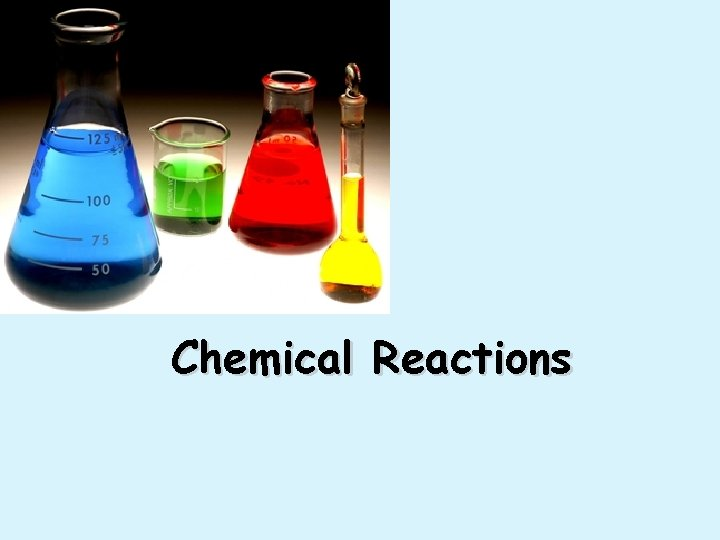Chemical ReactionsDescribing Chemical Reactions • Word equations • Chemical equations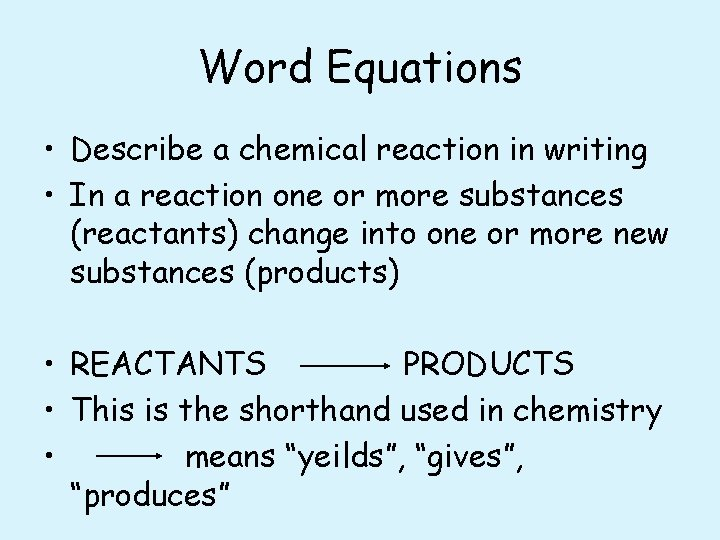Word Equations • Describe a chemical reaction in writing • In a reaction one or more substances (reactants) change into one or more new substances (products) • REACTANTS PRODUCTS • This is the shorthand used in chemistry • means “yeilds”, “gives”, “produces”Chemical Equations • Their Job: Depict the kind of reactants and products and their relative amounts in a reaction. 4 Al (s) + 3 O 2 (g) 2 Al 2 O 3 (s) • The numbers in the front are called stoichiometric coefficients • The letters (s), (g), and (l) are the physical states of compounds.Chemical Equations • This is quicker and more efficient than word equations • In chemical equations the arrow separates the formulas of the reactants from the formulas of the products • Example: Fe + O 2 Fe 2 O 3 • You should notice something wrong…Chemical Equations • Fe + O 2 Fe 2 O 3 • This is a skeleton equation • Shows formulas of reactants and products, but NOT the amounts of each • If you look at the reaction, the Law of Conservation of Matter is NOT obeyed! • Won’t use these much! • We MUST balance the equation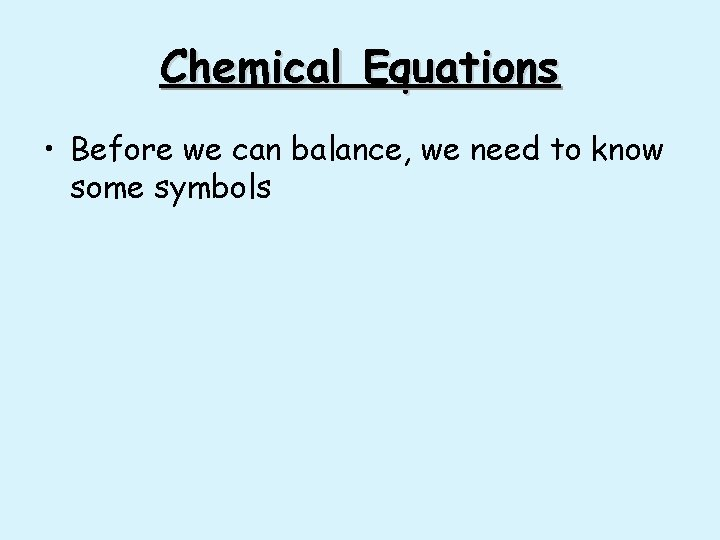Chemical Equations • Before we can balance, we need to know some symbolsSymbols used in Chemical Equations • the arrow separates the reactants from the products – Read as “reacts to form” or “yields” • The plus sign (+) = “and” • (s) after the formula = solid: Ag. Cl(s) • (g) after the formula = gas: CO 2 (g) • (l) after the formula = liquid: H 2 O (l)Symbols used in Equations § (aq) after the formula = dissolved in water, an aqueous solution: Na. Cl(aq) is a salt water solution § ↑ used after a product indicates a gas has been produced: H 2↑ § ↓ used after a product indicates a solid has been produced: Pb. I 2↓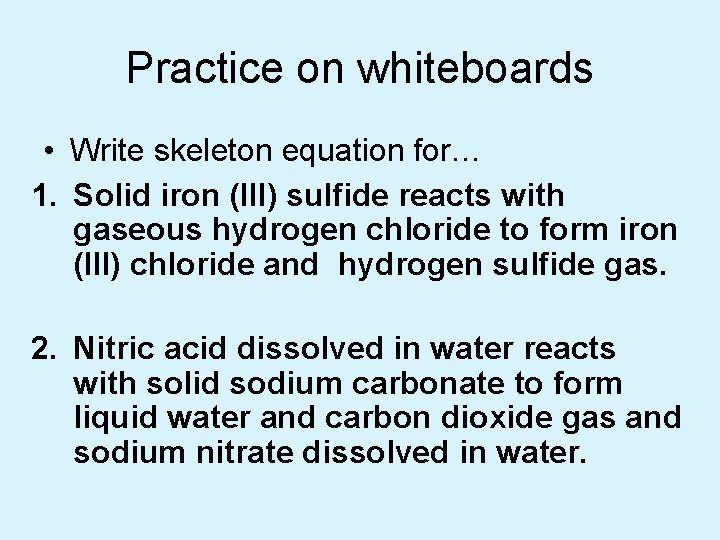Practice on whiteboards • Write skeleton equation for… 1. Solid iron (III) sulfide reacts with gaseous hydrogen chloride to form iron (III) chloride and hydrogen sulfide gas. 2. Nitric acid dissolved in water reacts with solid sodium carbonate to form liquid water and carbon dioxide gas and sodium nitrate dissolved in water.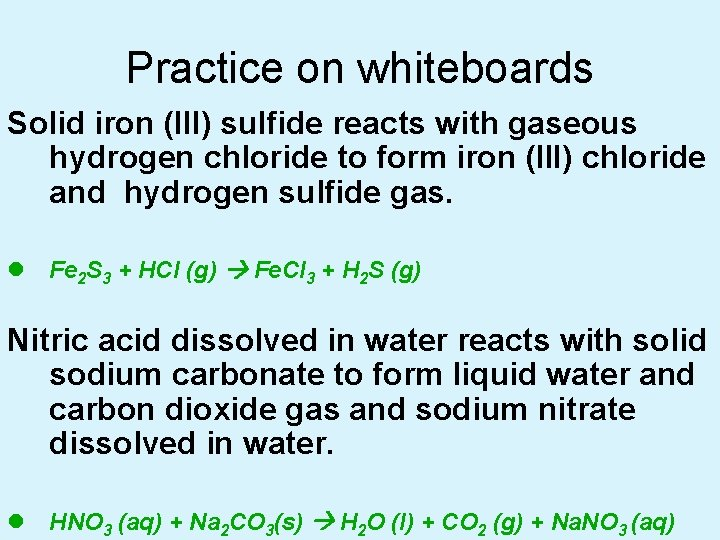Practice on whiteboards Solid iron (III) sulfide reacts with gaseous hydrogen chloride to form iron (III) chloride and hydrogen sulfide gas. l Fe 2 S 3 + HCl (g) Fe. Cl 3 + H 2 S (g) Nitric acid dissolved in water reacts with solid sodium carbonate to form liquid water and carbon dioxide gas and sodium nitrate dissolved in water. l HNO 3 (aq) + Na 2 CO 3(s) H 2 O (l) + CO 2 (g) + Na. NO 3 (aq)Now, write these in words… Fe(s) + O 2(g) ® Fe 2 O 3(s) Cu(s) + Ag. NO 3(aq) ® Ag(s) + Cu(NO 3)2(aq) NO 2 (g) N 2(g) + O 2(g)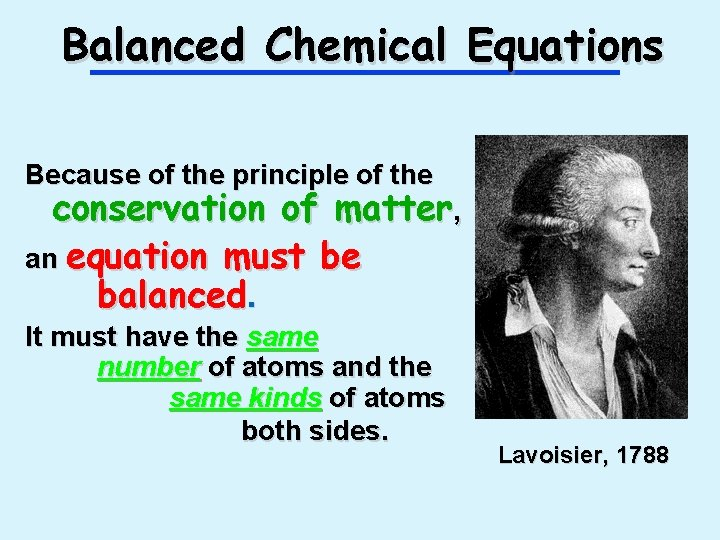Balanced Chemical Equations Because of the principle of the conservation of matter, an equation must be balanced. It must have the same number of atoms and the same kinds of atoms both sides. Lavoisier, 1788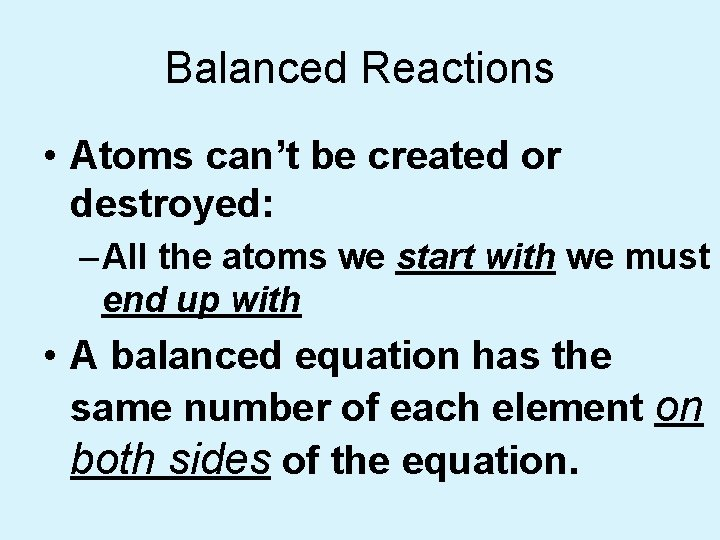Balanced Reactions • Atoms can’t be created or destroyed: – All the atoms we start with we must end up with • A balanced equation has the same number of each element on both sides of the equation.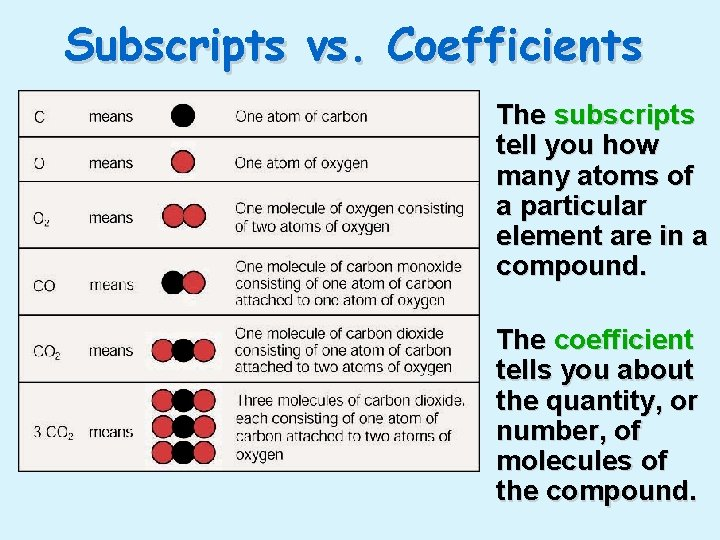Subscripts vs. Coefficients • The subscripts tell you how many atoms of a particular element are in a compound. • The coefficient tells you about the quantity, or number, of molecules of the compound.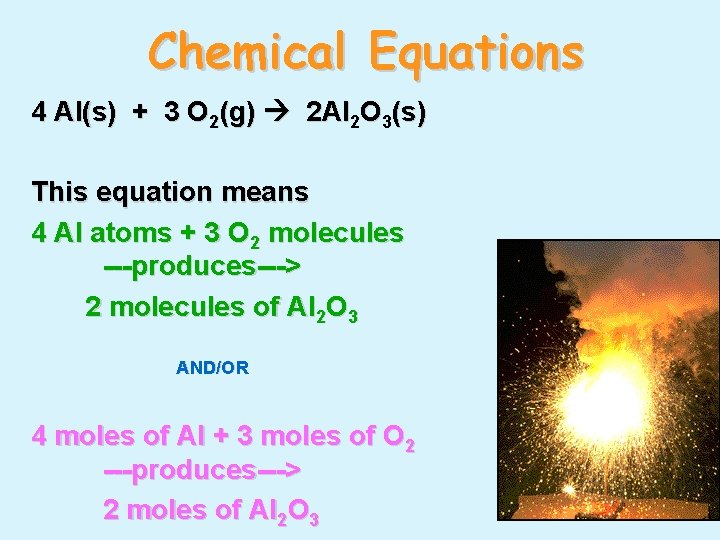Chemical Equations 4 Al(s) + 3 O 2(g) 2 Al 2 O 3(s) This equation means 4 Al atoms + 3 O 2 molecules ---produces---> 2 molecules of Al 2 O 3 AND/OR 4 moles of Al + 3 moles of O 2 ---produces---> 2 moles of Al 2 O 3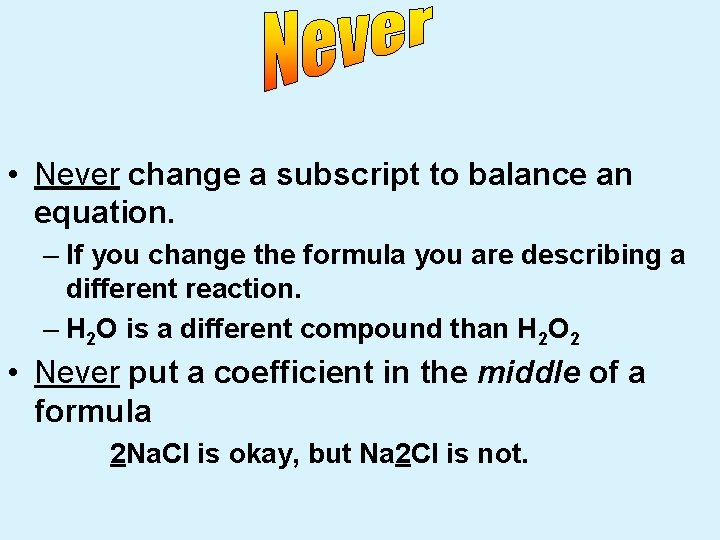• Never change a subscript to balance an equation. – If you change the formula you are describing a different reaction. – H 2 O is a different compound than H 2 O 2 • Never put a coefficient in the middle of a formula 2 Na. Cl is okay, but Na 2 Cl is not.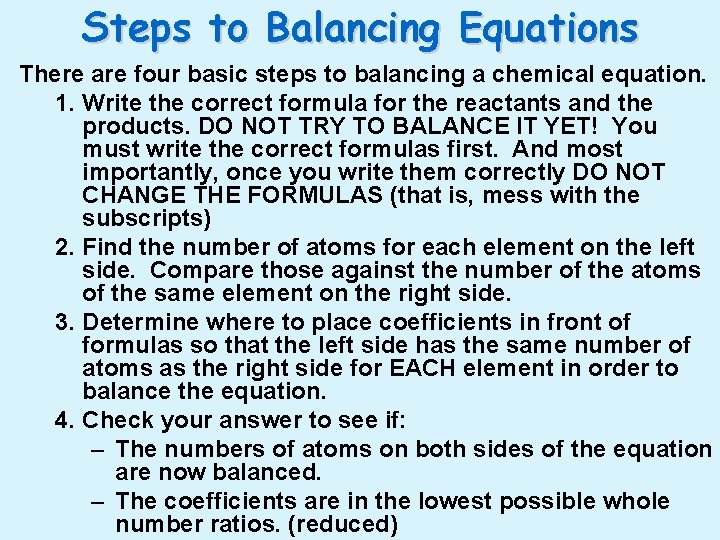Steps to Balancing Equations There are four basic steps to balancing a chemical equation. 1. Write the correct formula for the reactants and the products. DO NOT TRY TO BALANCE IT YET! You must write the correct formulas first. And most importantly, once you write them correctly DO NOT CHANGE THE FORMULAS (that is, mess with the subscripts) 2. Find the number of atoms for each element on the left side. Compare those against the number of the atoms of the same element on the right side. 3. Determine where to place coefficients in front of formulas so that the left side has the same number of atoms as the right side for EACH element in order to balance the equation. 4. Check your answer to see if: – The numbers of atoms on both sides of the equation are now balanced. – The coefficients are in the lowest possible whole number ratios. (reduced)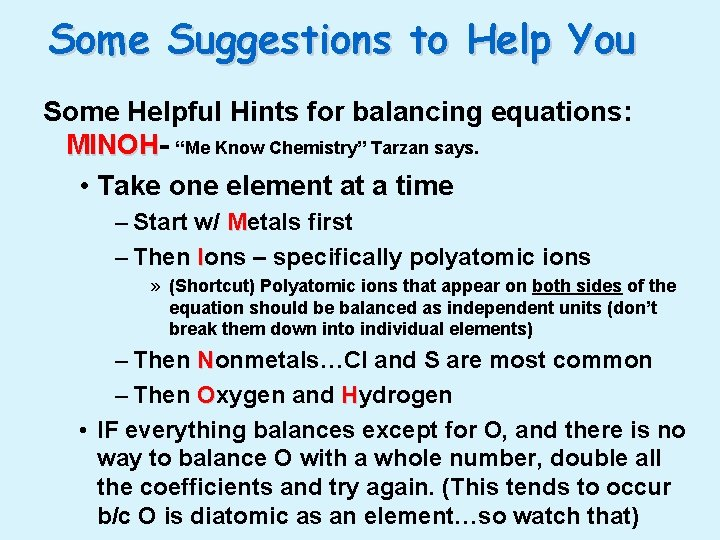Some Suggestions to Help You Some Helpful Hints for balancing equations: MINOH “Me Know Chemistry” Tarzan says. • Take one element at a time – Start w/ Metals first – Then Ions – specifically polyatomic ions » (Shortcut) Polyatomic ions that appear on both sides of the equation should be balanced as independent units (don’t break them down into individual elements) – Then Nonmetals…Cl and S are most common – Then Oxygen and Hydrogen • IF everything balances except for O, and there is no way to balance O with a whole number, double all the coefficients and try again. (This tends to occur b/c O is diatomic as an element…so watch that)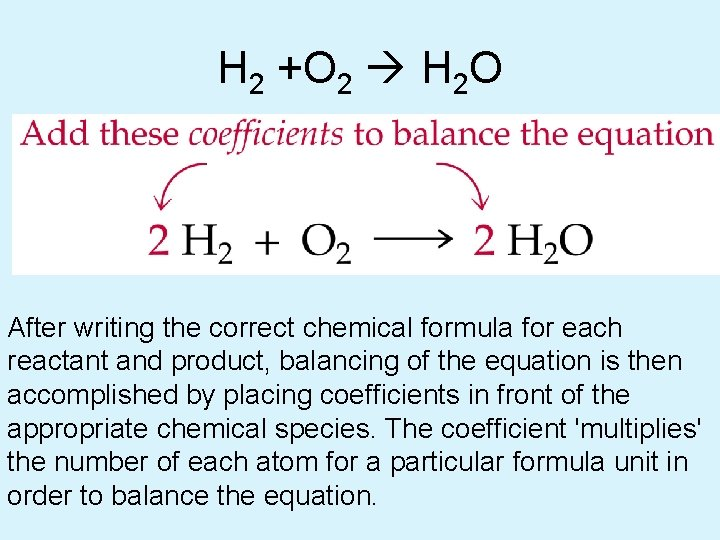H 2 +O 2 H 2 O After writing the correct chemical formula for each reactant and product, balancing of the equation is then accomplished by placing coefficients in front of the appropriate chemical species. The coefficient 'multiplies' the number of each atom for a particular formula unit in order to balance the equation.H 2 + O 2 H 2 O • When balancing chemical equations, coefficients are added in front of a particular species indicating multiple numbers of that species. Do not change subscripts since this actually changes the identity of the intended reactant or product• H 2 O is water • H 2 O 2 is hydrogen peroxide • They are very different!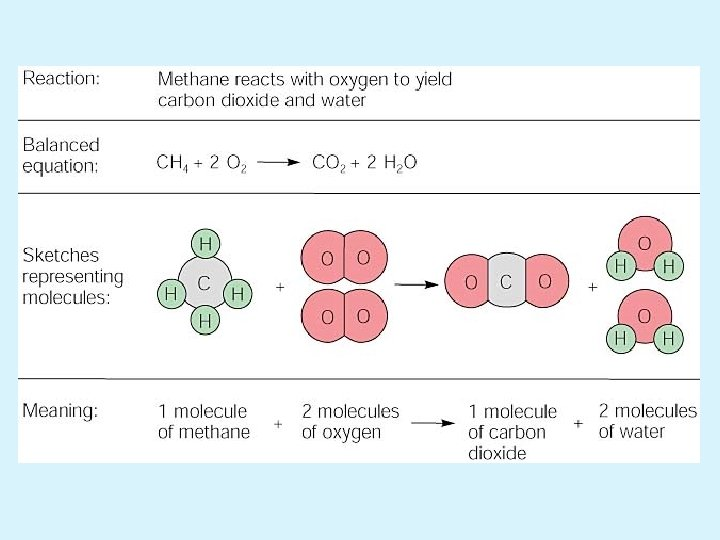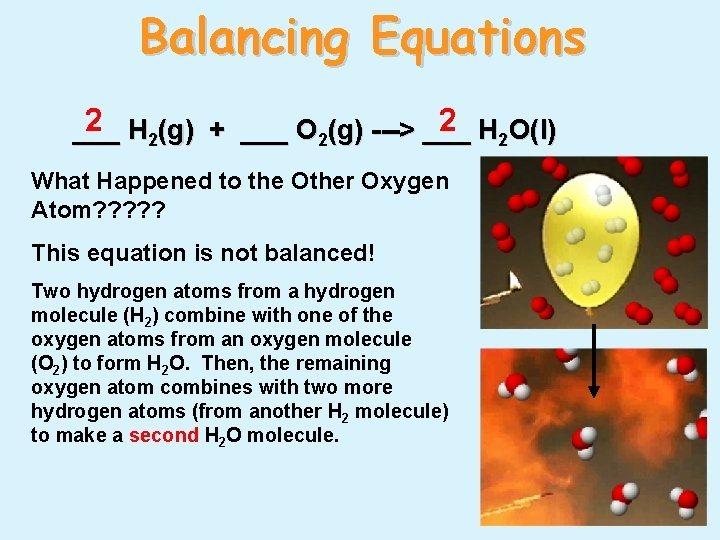Balancing Equations 2 H 2(g) + ___ O 2(g) ---> ___ 2 H 2 O(l) ___ What Happened to the Other Oxygen Atom? ? ? This equation is not balanced! Two hydrogen atoms from a hydrogen molecule (H 2) combine with one of the oxygen atoms from an oxygen molecule (O 2) to form H 2 O. Then, the remaining oxygen atom combines with two more hydrogen atoms (from another H 2 molecule) to make a second H 2 O molecule.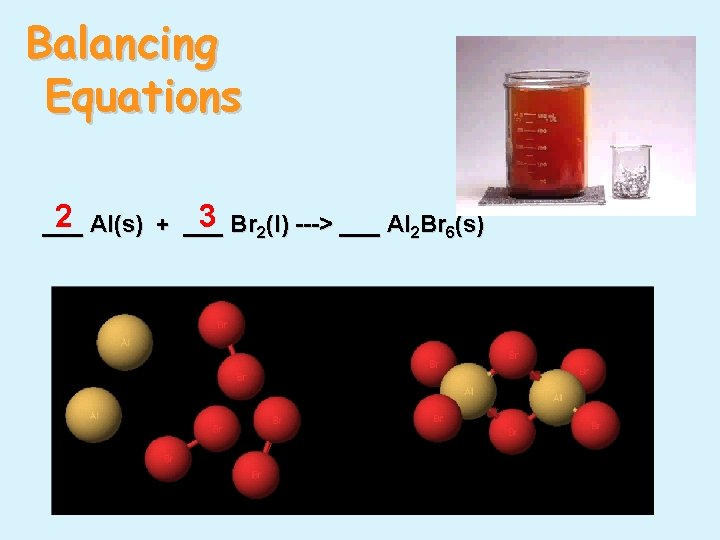Balancing Equations 2 Al(s) + ___ 3 Br 2(l) ---> ___ Al 2 Br 6(s) ___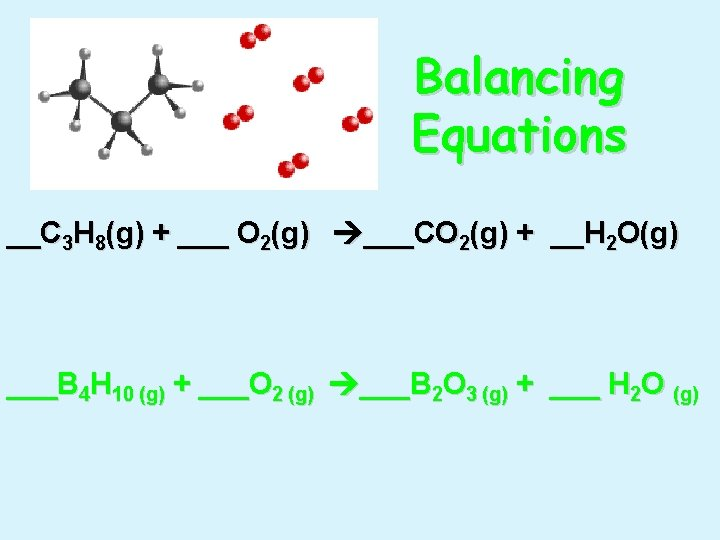Balancing Equations __C 3 H 8(g) + ___ O 2(g) ___CO 2(g) + __H 2 O(g) ___B 4 H 10 (g) + ___O 2 (g) ___B 2 O 3 (g) + ___ H 2 O (g)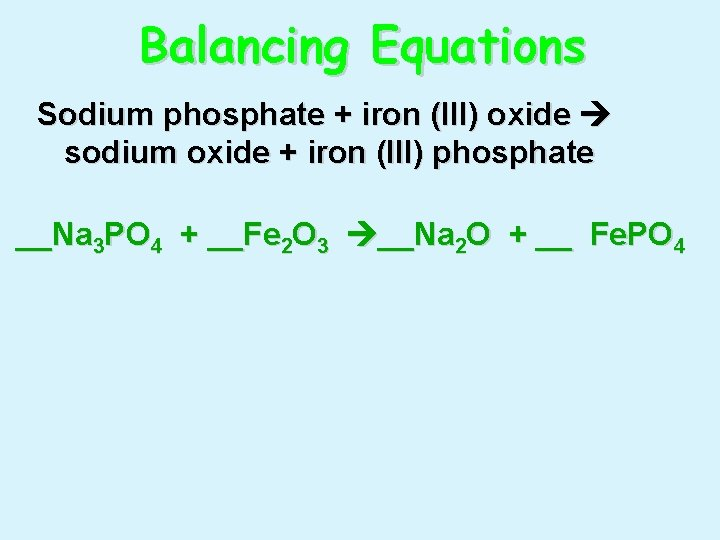Balancing Equations Sodium phosphate + iron (III) oxide sodium oxide + iron (III) phosphate __Na 3 PO 4 + __Fe 2 O 3 __Na 2 O + __ Fe. PO 4Practice Balancing Examples • _Ag. NO 3 + _Cu ® _Cu(NO 3)2 + _Ag • _Mg + _N 2 ® _Mg 3 N 2 • _P + _O 2 ® _P 4 O 10 • _Na + _H 2 O ® _H 2 + _Na. OH • _CH 4 + _O 2 ® _CO 2 + _H 2 O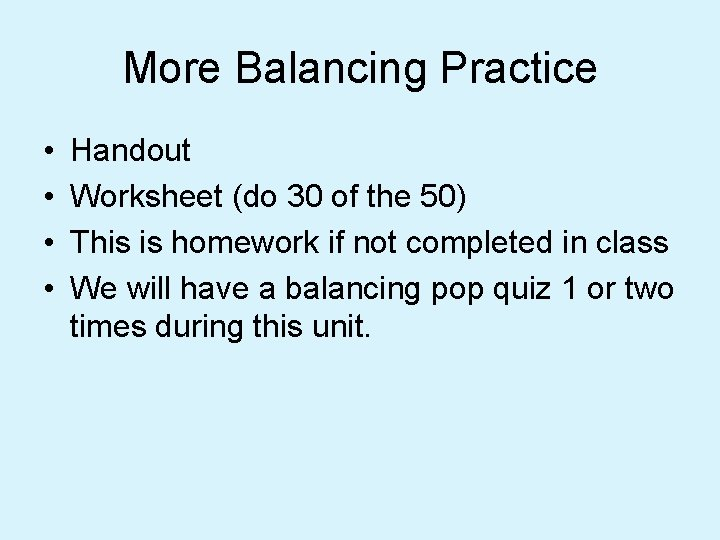More Balancing Practice • • Handout Worksheet (do 30 of the 50) This is homework if not completed in class We will have a balancing pop quiz 1 or two times during this unit.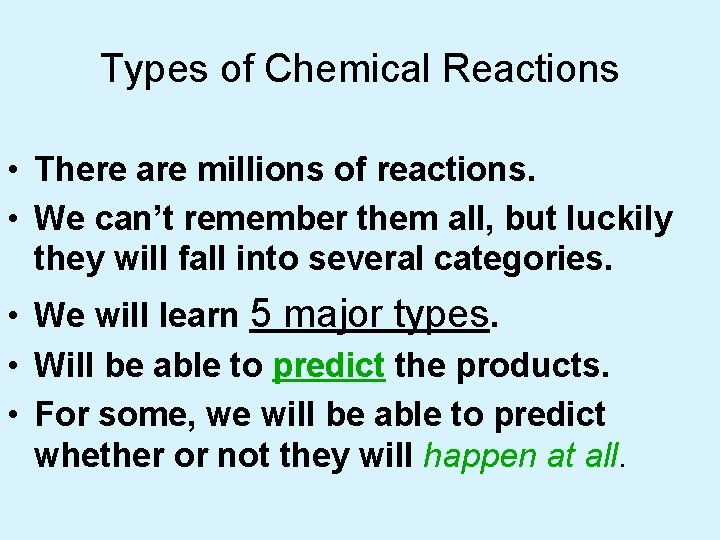Types of Chemical Reactions • There are millions of reactions. • We can’t remember them all, but luckily they will fall into several categories. • We will learn 5 major types. • Will be able to predict the products. • For some, we will be able to predict whether or not they will happen at all.Types of Reactions • There are five types of chemical reactions we will talk about: 1. 2. 3. 4. 5. • Synthesis reactions Decomposition reactions Single displacement reactions Double displacement reactions Combustion reactions You need to be able to identify the type of reaction and predict the product(s)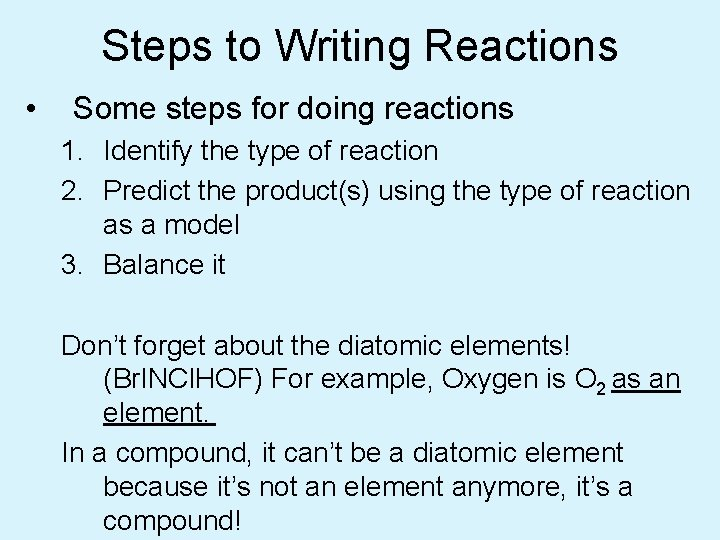Steps to Writing Reactions • Some steps for doing reactions 1. Identify the type of reaction 2. Predict the product(s) using the type of reaction as a model 3. Balance it Don’t forget about the diatomic elements! (Br. INCl. HOF) For example, Oxygen is O 2 as an element. In a compound, it can’t be a diatomic element because it’s not an element anymore, it’s a compound!1. Synthesis reactions • Synthesis reactions occur when two substances (generally elements) combine and form a compound. (Sometimes these are called combination or addition reactions. ) reactant + reactant 1 product • Basically: A + B AB • Example: 2 H 2 + O 2 2 H 2 O • Example: C + O 2 CO 2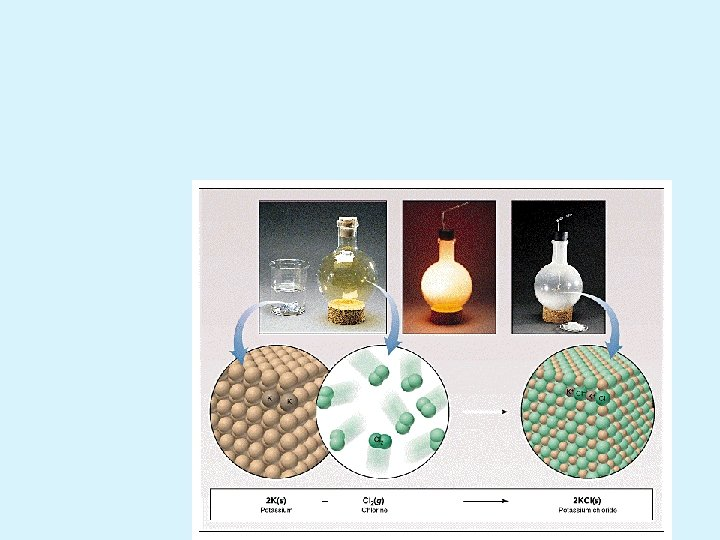Synthesis Reactions • Here is another example of a synthesis reaction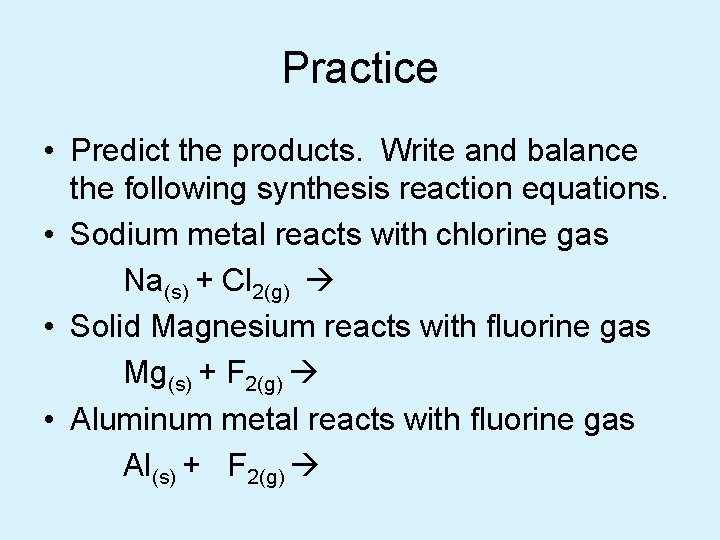Practice • Predict the products. Write and balance the following synthesis reaction equations. • Sodium metal reacts with chlorine gas Na(s) + Cl 2(g) • Solid Magnesium reacts with fluorine gas Mg(s) + F 2(g) • Aluminum metal reacts with fluorine gas Al(s) + F 2(g)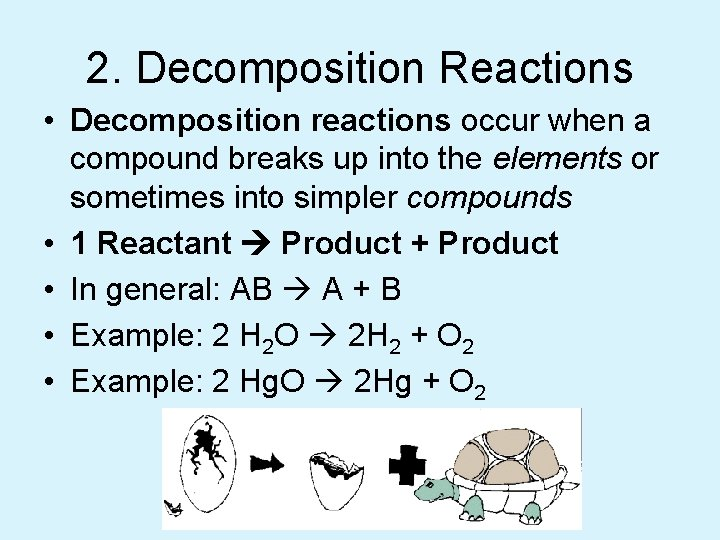2. Decomposition Reactions • Decomposition reactions occur when a compound breaks up into the elements or sometimes into simpler compounds • 1 Reactant Product + Product • In general: AB A + B • Example: 2 H 2 O 2 H 2 + O 2 • Example: 2 Hg. O 2 Hg + O 2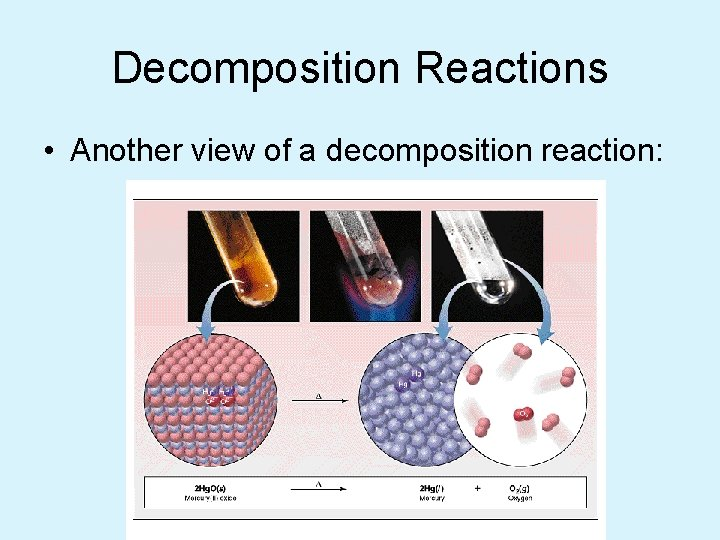Decomposition Reactions • Another view of a decomposition reaction: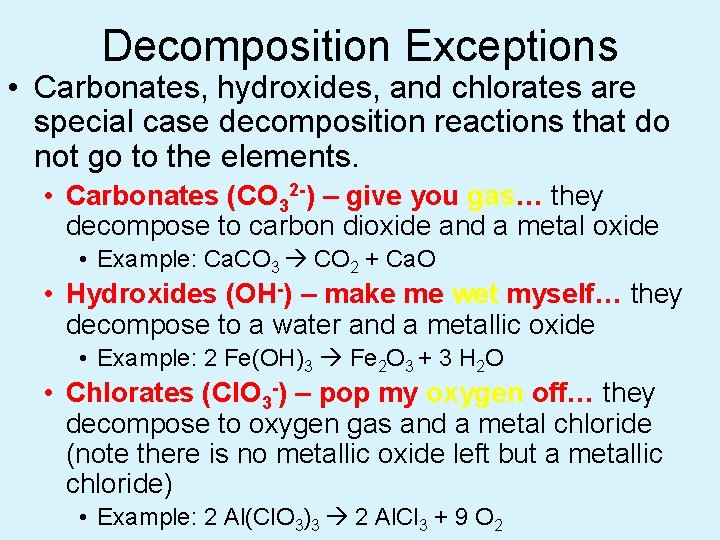Decomposition Exceptions • Carbonates, hydroxides, and chlorates are special case decomposition reactions that do not go to the elements. • Carbonates (CO 32 -) – give you gas… they decompose to carbon dioxide and a metal oxide • Example: Ca. CO 3 CO 2 + Ca. O • Hydroxides (OH-) – make me wet myself… they decompose to a water and a metallic oxide • Example: 2 Fe(OH)3 Fe 2 O 3 + 3 H 2 O • Chlorates (Cl. O 3 -) – pop my oxygen off… they decompose to oxygen gas and a metal chloride (note there is no metallic oxide left but a metallic chloride) • Example: 2 Al(Cl. O 3)3 2 Al. Cl 3 + 9 O 2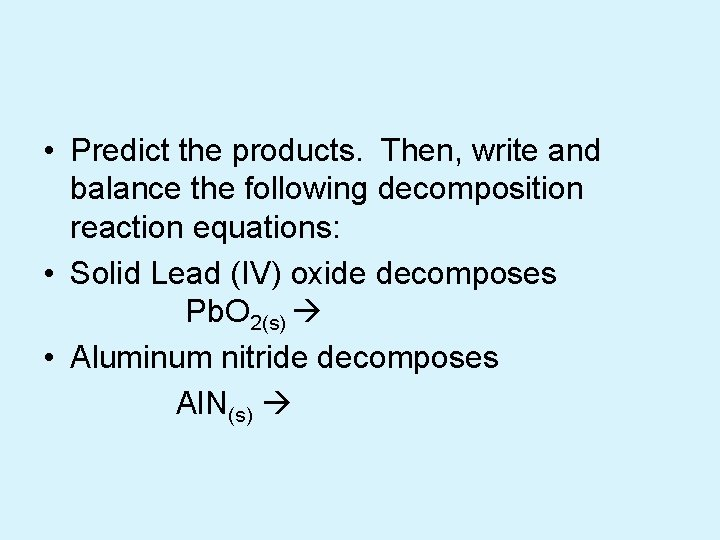Practice • Predict the products. Then, write and balance the following decomposition reaction equations: • Solid Lead (IV) oxide decomposes Pb. O 2(s) • Aluminum nitride decomposes Al. N(s)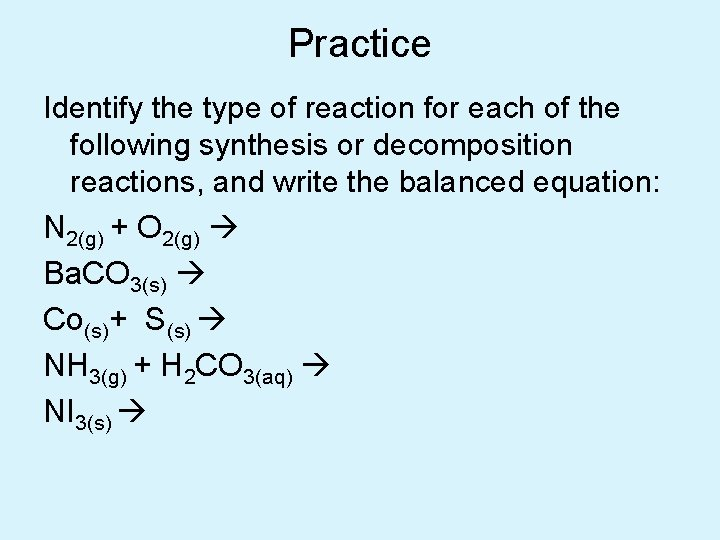Practice Identify the type of reaction for each of the following synthesis or decomposition reactions, and write the balanced equation: N 2(g) + O 2(g) Nitrogen monoxide Ba. CO 3(s) Co(s)+ S(s) (make Co be +3) NH 3(g) + H 2 CO 3(aq) NI 3(s)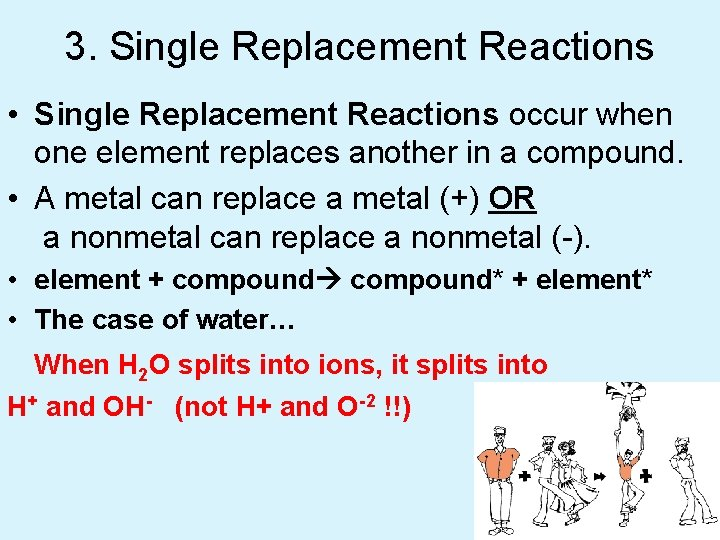3. Single Replacement Reactions • Single Replacement Reactions occur when one element replaces another in a compound. • A metal can replace a metal (+) OR a nonmetal can replace a nonmetal (-). • element + compound* + element* • The case of water… When H 2 O splits into ions, it splits into H+ and OH- (not H+ and O-2 !!)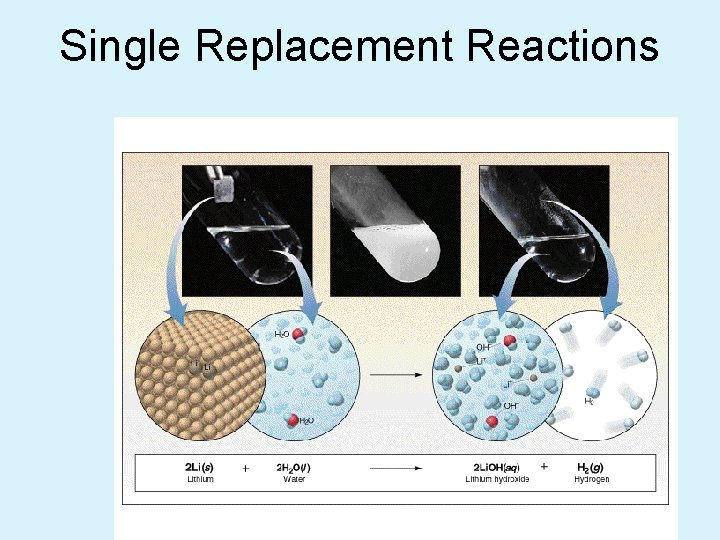Single Replacement Reactions • Another view: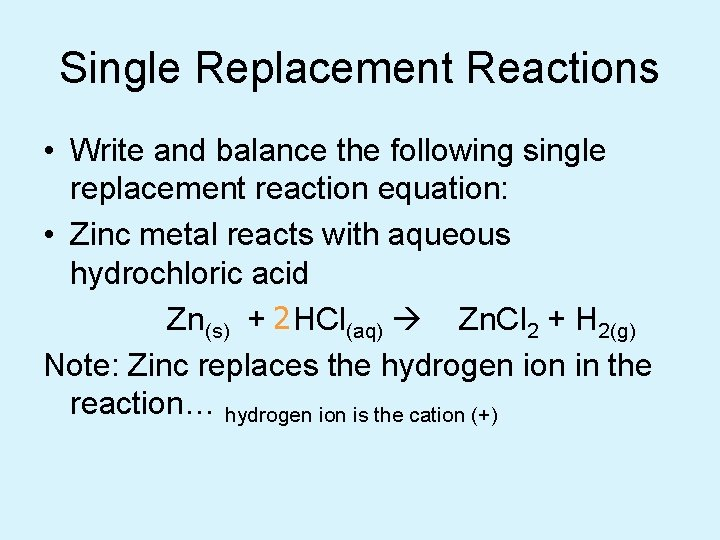Single Replacement Reactions • Write and balance the following single replacement reaction equation: • Zinc metal reacts with aqueous hydrochloric acid Zn(s) + 2 HCl(aq) Zn. Cl 2 + H 2(g) Note: Zinc replaces the hydrogen ion in the reaction… hydrogen ion is the cation (+)Single Replacement • We can even tell whether or not a single replacement reaction will happen: – Some chemicals are more “active” than others – More active replaces less active • There is a list called the Activity Series of Metals • Higher on the list replaces lower.The Activity Series of the Metals Higher activity Lower activity Lithium Potassium Calcium Sodium Magnesium Aluminum Zinc Chromium Iron Nickel Lead Hydrogen Bismuth Copper Mercury Silver Platinum Gold 1) Metals can replace other metals provided that they are above the metal that they are trying to replace. 2) Metals above hydrogen can replace hydrogen in acids. 3) Metals from sodium upward can replace hydrogen in water.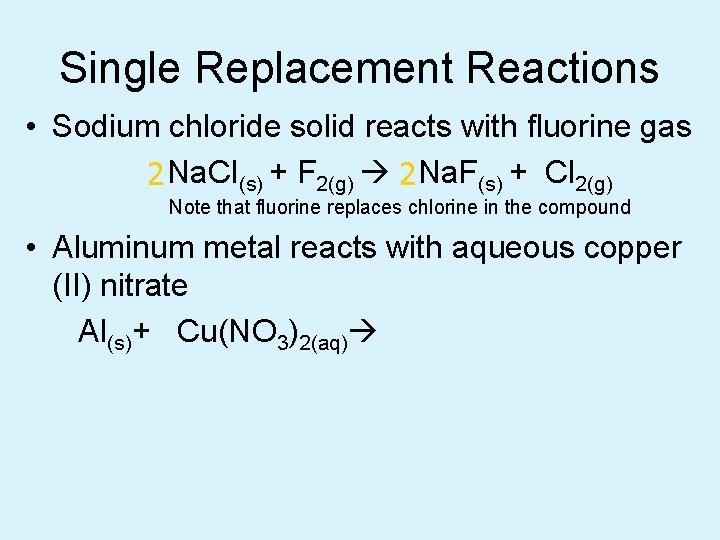Single Replacement Reactions • Sodium chloride solid reacts with fluorine gas 2 Na. Cl(s) + F 2(g) 2 Na. F(s) + Cl 2(g) Note that fluorine replaces chlorine in the compound • Aluminum metal reacts with aqueous copper (II) nitrate Al(s)+ Cu(NO 3)2(aq)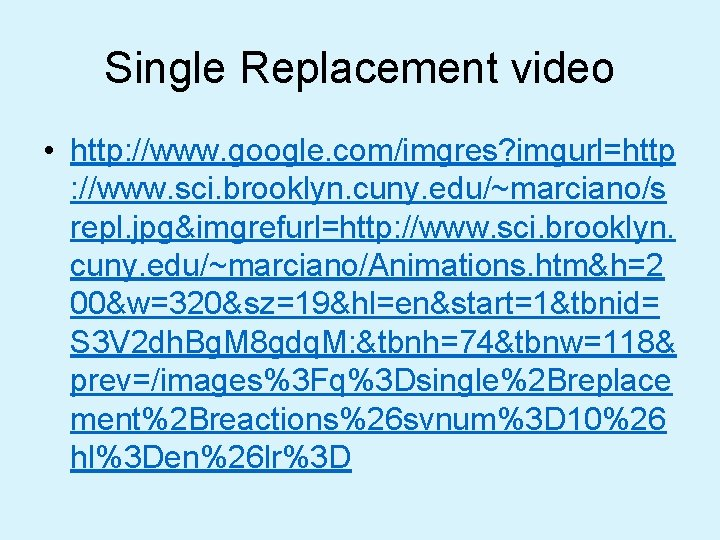Single Replacement video • http: //www. google. com/imgres? imgurl=http : //www. sci. brooklyn. cuny. edu/~marciano/s repl. jpg&imgrefurl=http: //www. sci. brooklyn. cuny. edu/~marciano/Animations. htm&h=2 00&w=320&sz=19&hl=en&start=1&tbnid= S 3 V 2 dh. Bg. M 8 gdq. M: &tbnh=74&tbnw=118& prev=/images%3 Fq%3 Dsingle%2 Breplace ment%2 Breactions%26 svnum%3 D 10%26 hl%3 Den%26 lr%3 D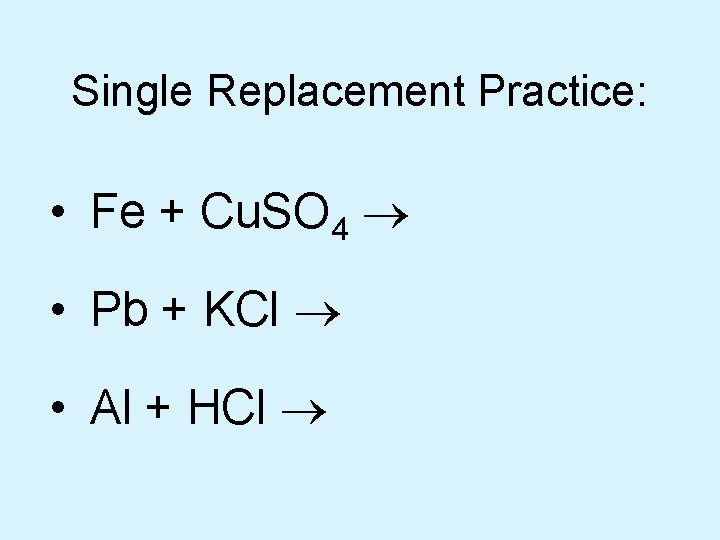Single Replacement Practice: • Fe + Cu. SO 4 ® • Pb + KCl ® • Al + HCl ®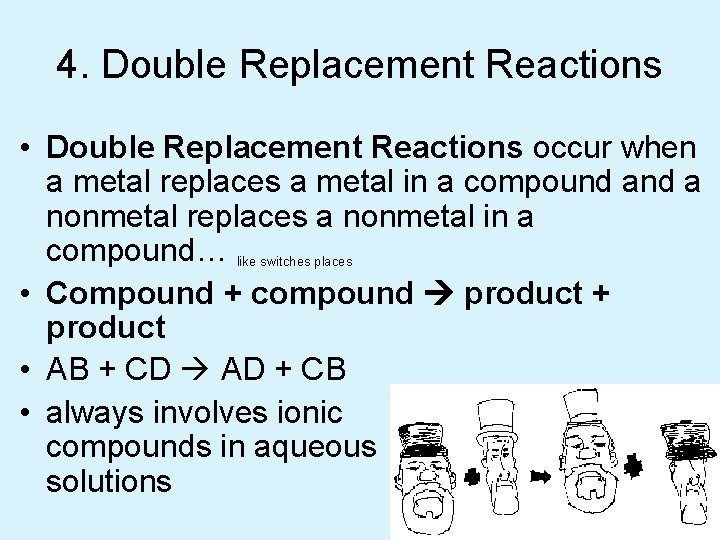4. Double Replacement Reactions • Double Replacement Reactions occur when a metal replaces a metal in a compound a nonmetal replaces a nonmetal in a compound… like switches places • Compound + compound product + product • AB + CD AD + CB • always involves ionic compounds in aqueous solutions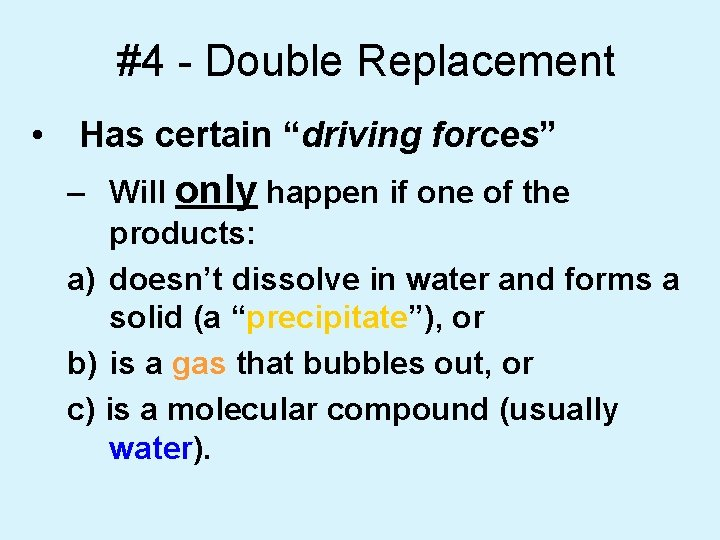#4 - Double Replacement • Has certain “driving forces” – Will only happen if one of the products: a) doesn’t dissolve in water and forms a solid (a “precipitate”), or b) is a gas that bubbles out, or c) is a molecular compound (usually water).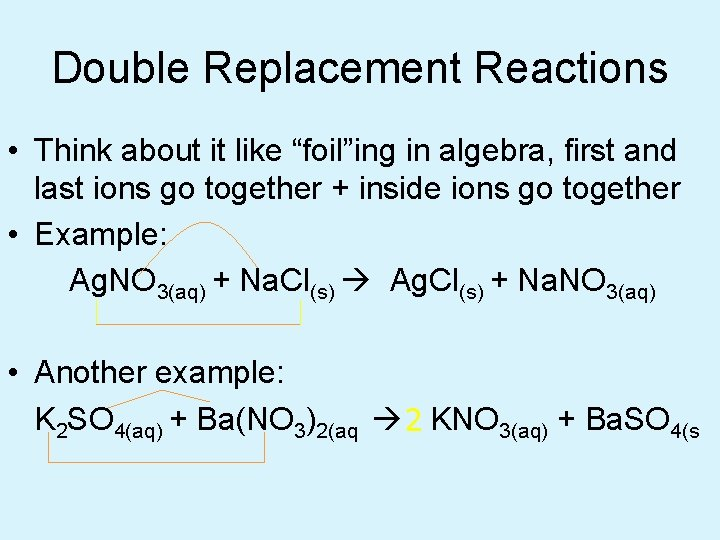Double Replacement Reactions • Think about it like “foil”ing in algebra, first and last ions go together + inside ions go together • Example: Ag. NO 3(aq) + Na. Cl(s) Ag. Cl(s) + Na. NO 3(aq) • Another example: K 2 SO 4(aq) + Ba(NO 3)2(aq) 2 KNO 3(aq) + Ba. SO 4(s)Double Replacement Video • http: //www. google. com/imgres? imgurl=http : //www. sci. brooklyn. cuny. edu/~marciano/s repl. jpg&imgrefurl=http: //www. sci. brooklyn. cuny. edu/~marciano/Animations. htm&h=2 00&w=320&sz=19&hl=en&start=1&tbnid= S 3 V 2 dh. Bg. M 8 gdq. M: &tbnh=74&tbnw=118& prev=/images%3 Fq%3 Dsingle%2 Breplace ment%2 Breactions%26 svnum%3 D 10%26 hl%3 Den%26 lr%3 DPractice • Predict the products. Balance the equation 1. HCl(aq) + Ag. NO 3(aq) 2. Ca. Cl 2(aq) + Na 3 PO 4(aq) 3. Pb(NO 3)2(aq) + Ba. Cl 2(aq) 4. Fe. Cl 3(aq) + Na. OH(aq) 5. H 2 SO 4(aq) + Na. OH(aq) 6. KOH(aq) + Cu. SO 4(aq)How to recognize which type • Look at the reactants: E + E = Combination/synthesis C = Decomposition E+C = Single replacement C + C = Double replacement5. Combustion Reactions • Combustion reactions occur when a hydrocarbon reacts with oxygen gas. • This is also called burning!!! In order to burn something you need the 3 things in the “fire triangle”: 1) A Fuel (hydrocarbon) 2) Oxygen to burn it with 3) Something to ignite the reaction (spark)Combustion Reactions • In general: Cx. Hy + O 2 CO 2 + H 2 O • Products in complete combustion are ALWAYS carbon dioxide and water. • Products of incomplete combustion can be carbon and carbon monoxide (Poisonous) • Many times heat and light are given off as by-products of the reaction • Combustion is used to heat homes and run automobiles (octane, as in gasoline, is C 8 H 18)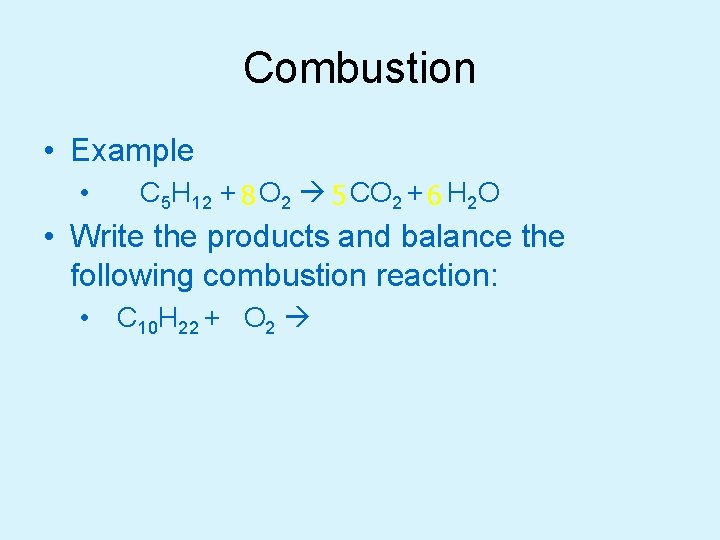Combustion • Example • C 5 H 12 + 8 O 2 5 CO 2 + 6 H 2 O • Write the products and balance the following combustion reaction: • C 10 H 22 + O 2Mixed Practice • 1. 2. 3. 4. 5. State the type, predict the products, and balance the following reactions: Ba. Cl 2 + H 2 SO 4 C 6 H 12 + O 2 Zn + Cu. SO 4 Cs + Br 2 Fe. CO 3Total Ionic Equations • Once you write the molecular equation (synthesis, decomposition, etc. ), you should check for reactants and products that are soluble or insoluble. • We usually assume the reaction is in water • We can use a solubility table to tell us what compounds dissolve in water. • If the compound is soluble (does dissolve in water), then splits the compound into its component ions • If the compound is insoluble (does NOT dissolve in water), then it remains as a compound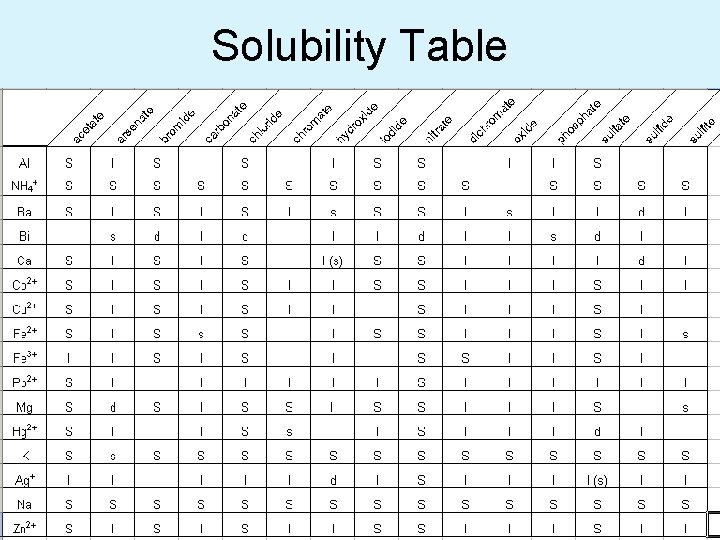Solubility Table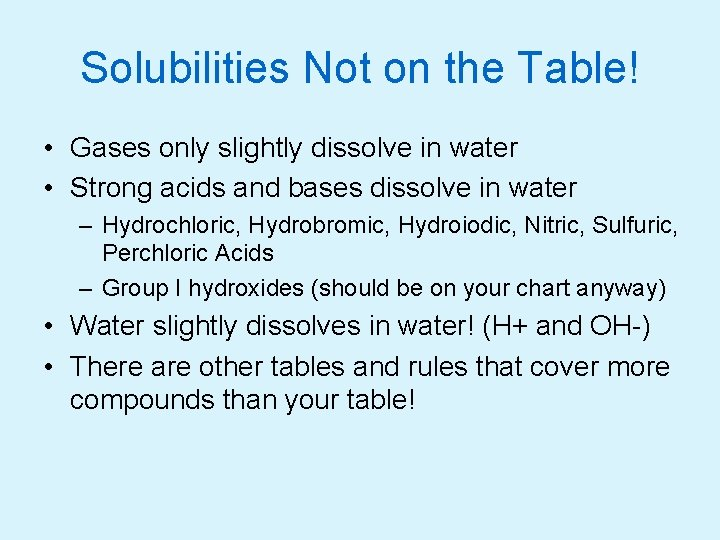Solubilities Not on the Table! • Gases only slightly dissolve in water • Strong acids and bases dissolve in water – Hydrochloric, Hydrobromic, Hydroiodic, Nitric, Sulfuric, Perchloric Acids – Group I hydroxides (should be on your chart anyway) • Water slightly dissolves in water! (H+ and OH-) • There are other tables and rules that cover more compounds than your table!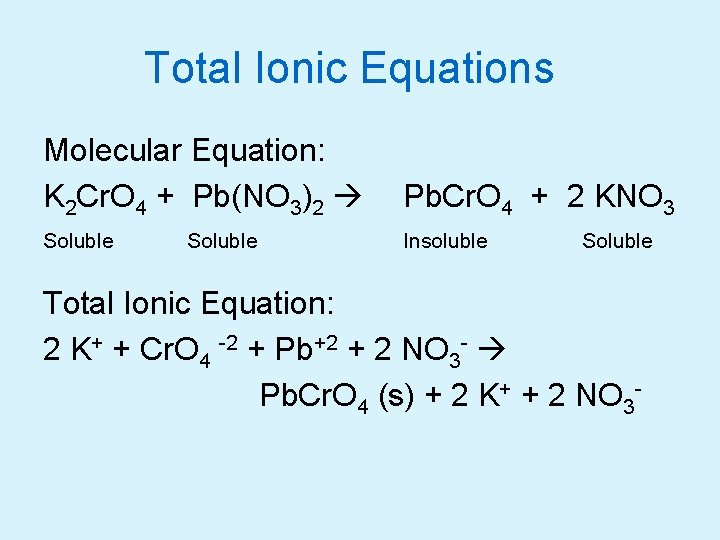Total Ionic Equations Molecular Equation: K 2 Cr. O 4 + Pb(NO 3)2 Pb. Cr. O 4 + 2 KNO 3 Soluble Insoluble Soluble Total Ionic Equation: 2 K+ + Cr. O 4 -2 + Pb+2 + 2 NO 3 - Pb. Cr. O 4 (s) + 2 K+ + 2 NO 3 -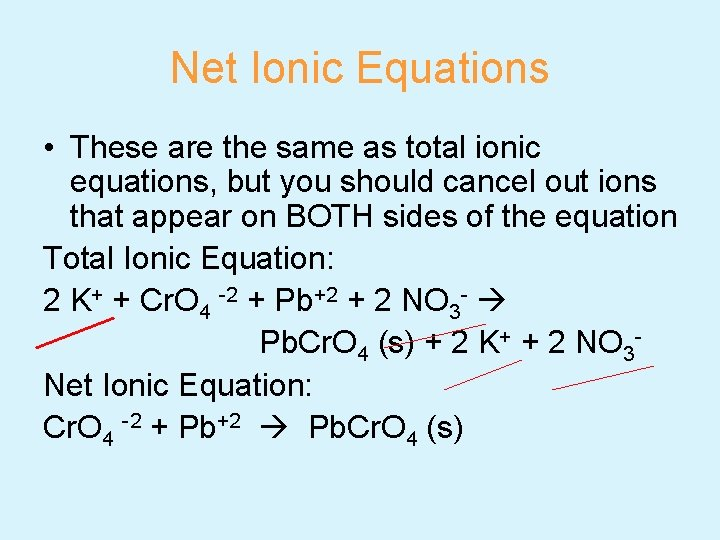Net Ionic Equations • These are the same as total ionic equations, but you should cancel out ions that appear on BOTH sides of the equation Total Ionic Equation: 2 K+ + Cr. O 4 -2 + Pb+2 + 2 NO 3 - Pb. Cr. O 4 (s) + 2 K+ + 2 NO 3 Net Ionic Equation: Cr. O 4 -2 + Pb+2 Pb. Cr. O 4 (s)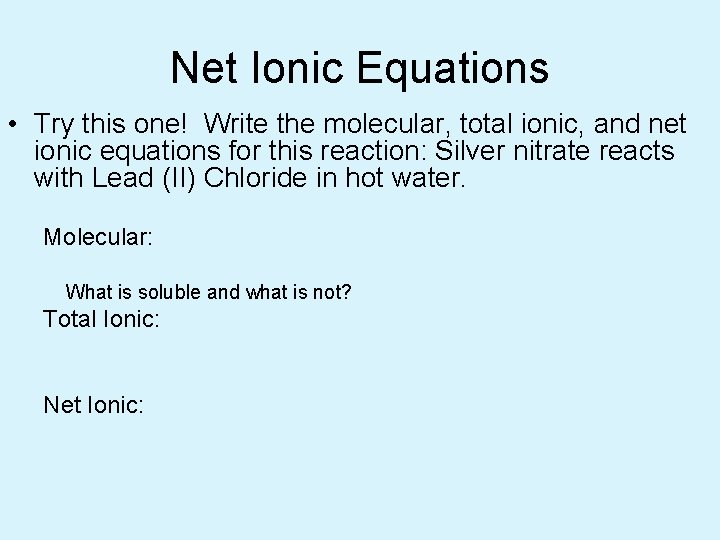Net Ionic Equations • Try this one! Write the molecular, total ionic, and net ionic equations for this reaction: Silver nitrate reacts with Lead (II) Chloride in hot water. Molecular: What is soluble and what is not? Total Ionic: Net Ionic: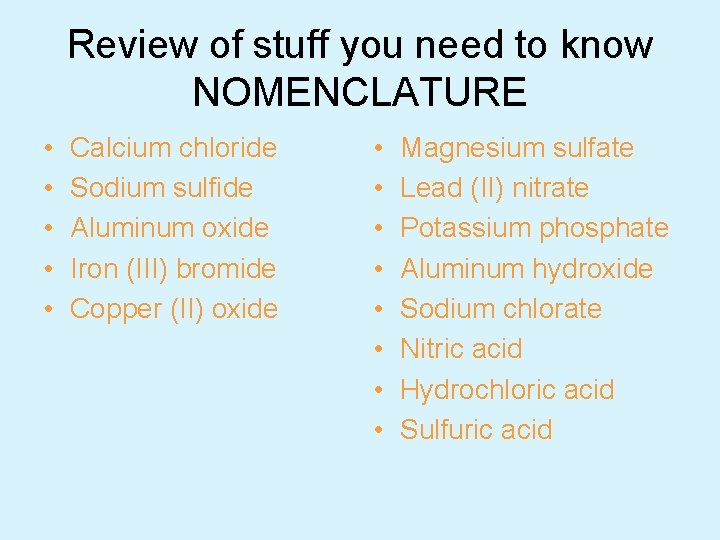Review of stuff you need to know NOMENCLATURE • • • Calcium chloride Sodium sulfide Aluminum oxide Iron (III) bromide Copper (II) oxide • • Magnesium sulfate Lead (II) nitrate Potassium phosphate Aluminum hydroxide Sodium chlorate Nitric acid Hydrochloric acid Sulfuric acid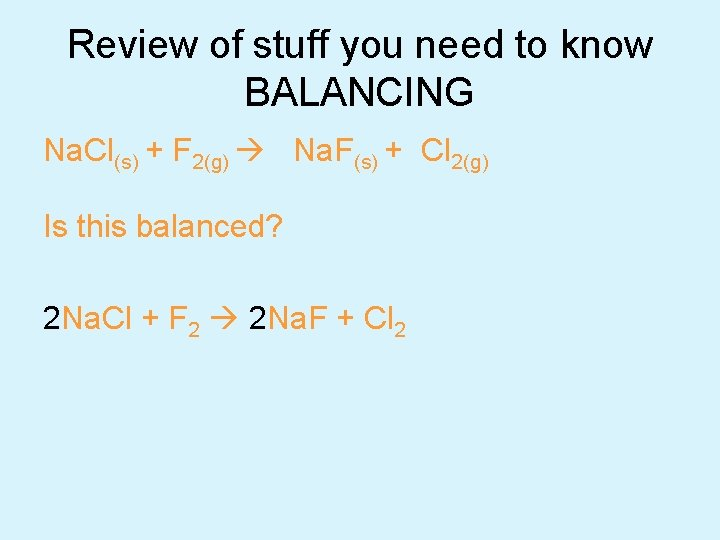Review of stuff you need to know BALANCING Na. Cl(s) + F 2(g) Na. F(s) + Cl 2(g) Is this balanced? 2 Na. Cl + F 2 2 Na. F + Cl 2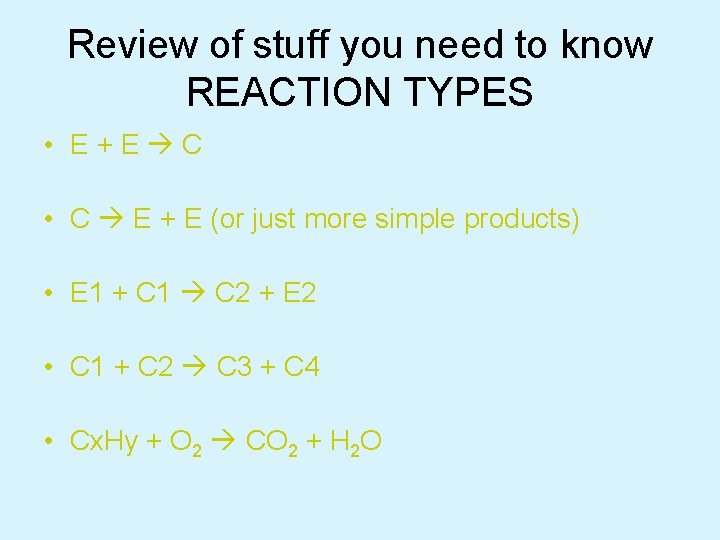Review of stuff you need to know REACTION TYPES • E+E C • C E + E (or just more simple products) • E 1 + C 1 C 2 + E 2 • C 1 + C 2 C 3 + C 4 • Cx. Hy + O 2 CO 2 + H 2 O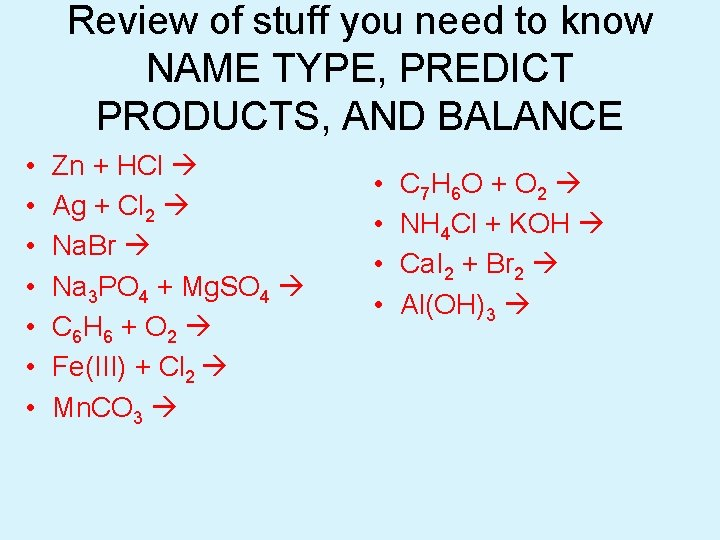Review of stuff you need to know NAME TYPE, PREDICT PRODUCTS, AND BALANCE • • Zn + HCl Ag + Cl 2 Na. Br Na 3 PO 4 + Mg. SO 4 C 6 H 6 + O 2 Fe(III) + Cl 2 Mn. CO 3 • • C 7 H 6 O + O 2 NH 4 Cl + KOH Ca. I 2 + Br 2 Al(OH)3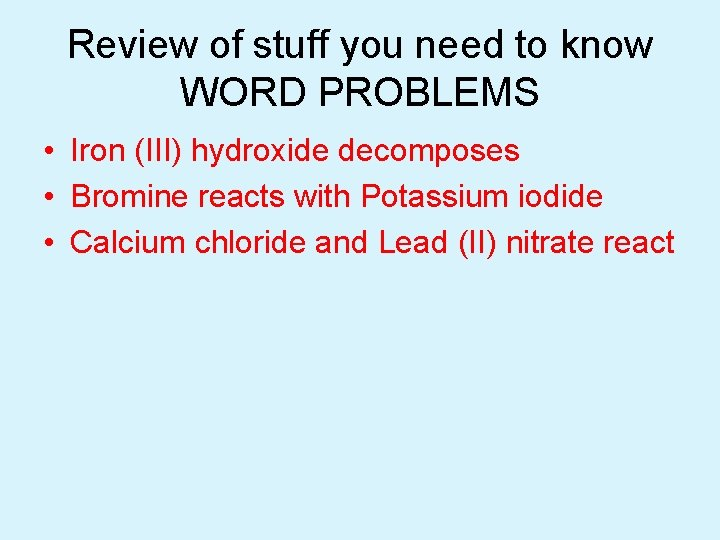Review of stuff you need to know WORD PROBLEMS • Iron (III) hydroxide decomposes • Bromine reacts with Potassium iodide • Calcium chloride and Lead (II) nitrate react